Practice using the FOIL system in this quiz.

# Algebra Intermediate - Factoring Trinomials [First term is other than 1]

This Math quiz is called 'Algebra Intermediate - Factoring Trinomials [First term is other than 1]' and it has been written by teachers to help you if you are studying the subject at middle school. Playing educational quizzes is a fabulous way to learn if you are in the 6th, 7th or 8th grade - aged 11 to 14.

It costs only \$12.50 per month to play this quiz and over 3,500 others that help you with your school work. You can subscribe on the page at Join Us

If you have not already taken the quiz on Factoring Trinomials [First term is “1”] then please go and check that quiz out first. It is a stepping stone to this more advanced quiz in which we will be dealing with the first term being a number other than the number “1”.

Once again, when you hear the word “factoring” in math, you know that you will be doing multiplication. You also know that when factoring trinomials you are essentially working a problem backwards, i.e., you are looking for the two binomial factors that will produce the trinomial problem. In order to accomplish this, you will need to be familiar with the FOIL system.

As a quick refresher, the FOIL system stands for working your problem by multiplying: F: the first terms from each factor, O: then the outer terms from each factor, I: next is the inner term from each factor and, finally, L: the last term of each factor. To see an example of how this works, please check out the quiz Factoring Trinomials [First term is “1”] mentioned above as it will provide you with more details on using the FOIL system.

Go straight to Quiz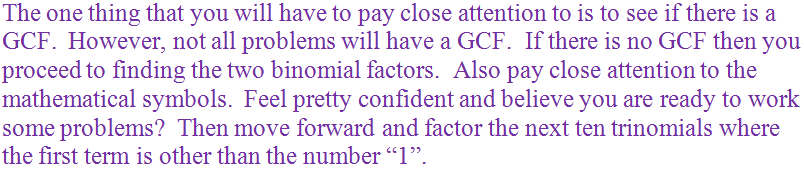1.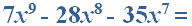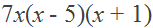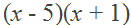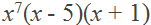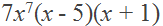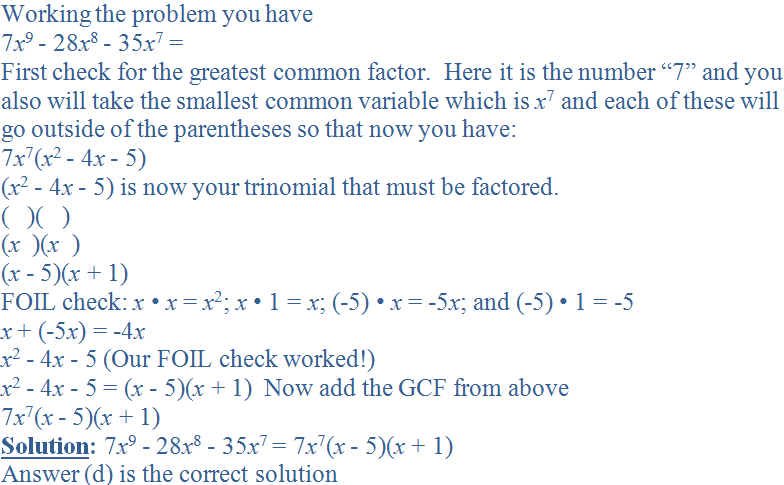2.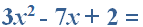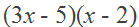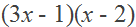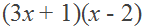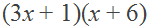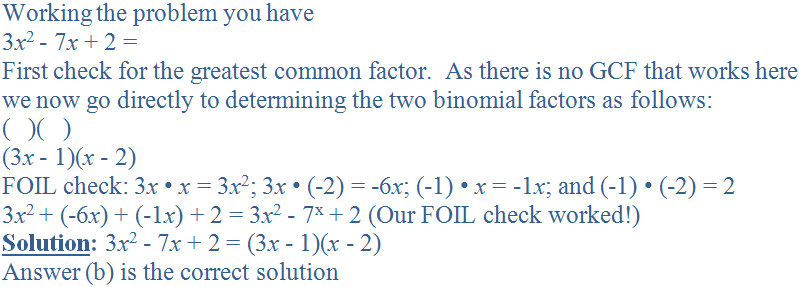3.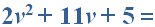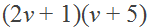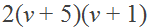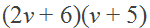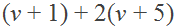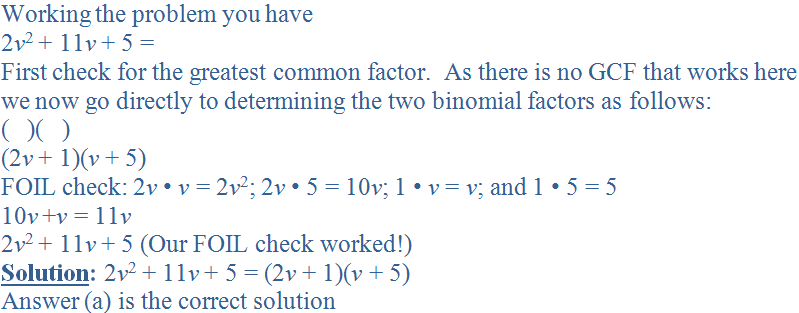4.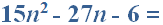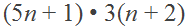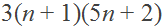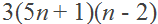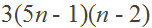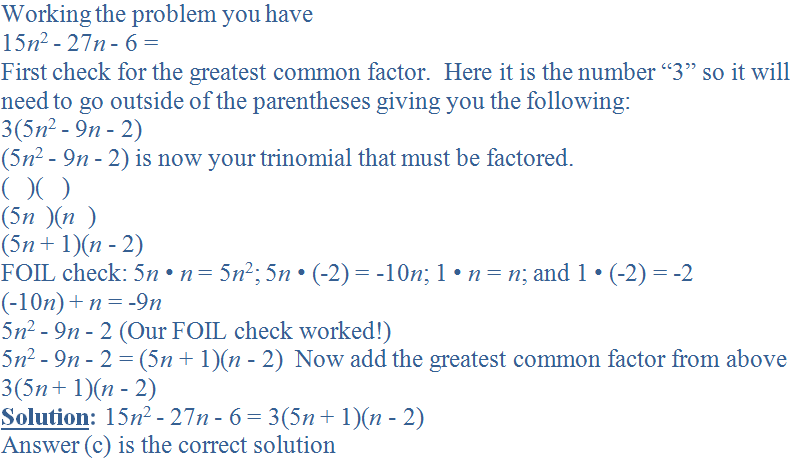5.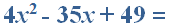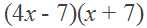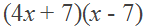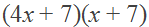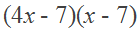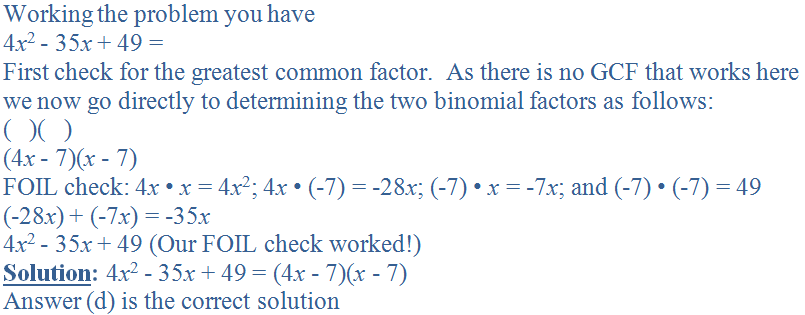6.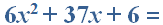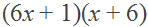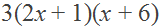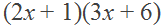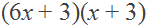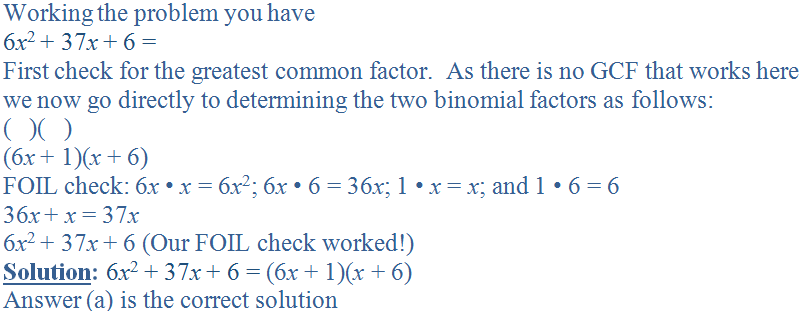7.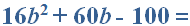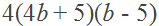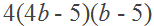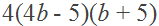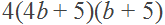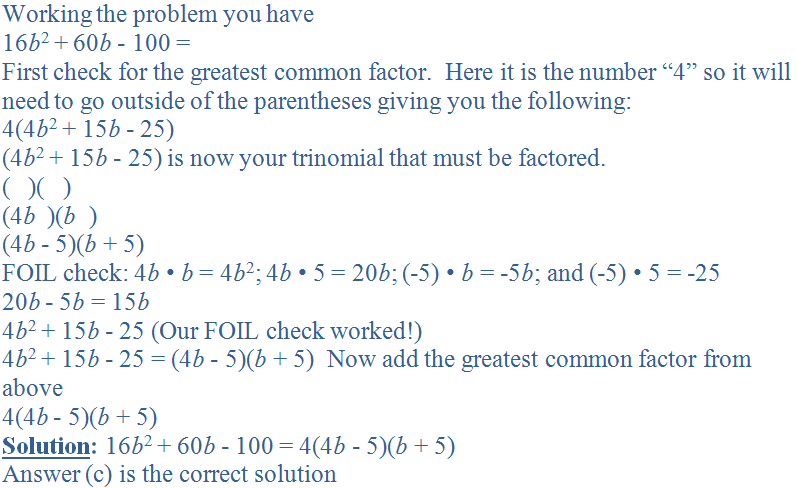8.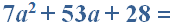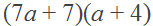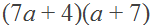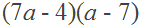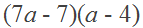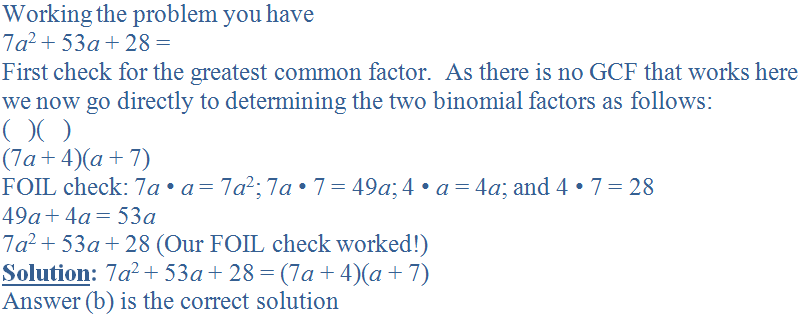9.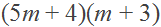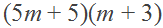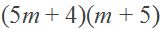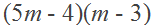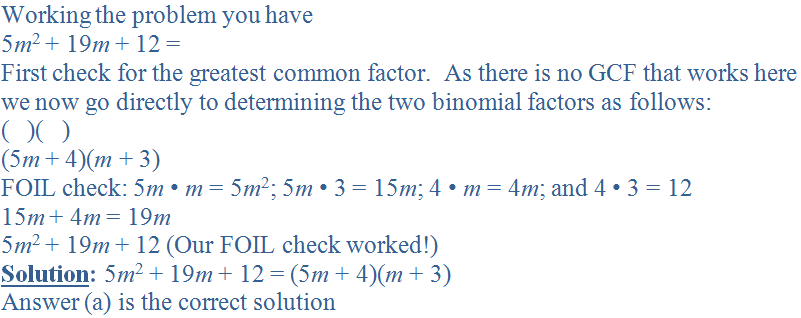10.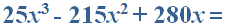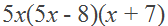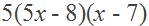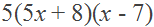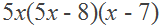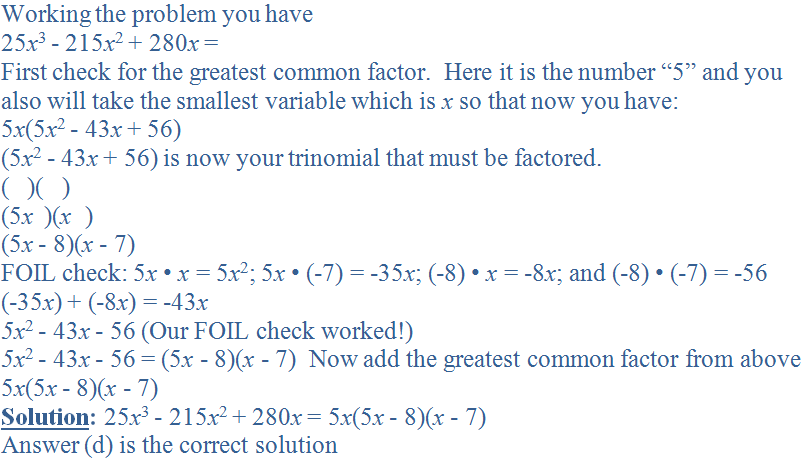Author:  Christine G. Broome